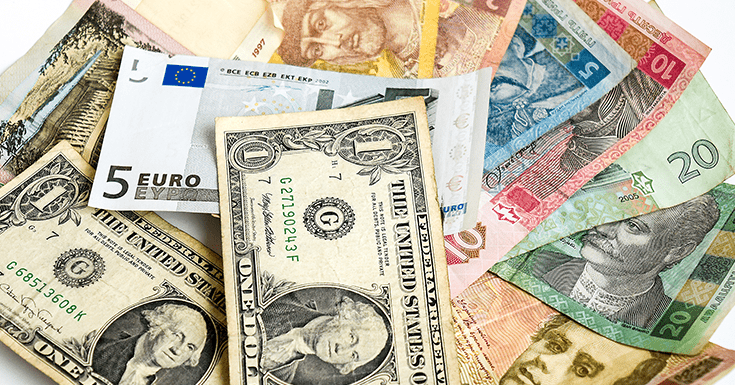# How to Easily Calculate Cross Currency RatesHow to Easily Calculate Cross Currency Rates
July 31, 2017

When executing trades between the major currencies, the process is usually quick and easy. However, when your trades involve currencies that are less common, transactions are not always easy because the rates are not always quoted making it difficult to trade exotic currencies without establishing an appropriate rate of exchange. This rate is called the cross currency rate and in this article we will show you how manually calculate it.

A cross exchange rate is mostly used when the currency pair being traded does not involve the US Dollar. The reason behind it is that conventionally if one wanted to convert a non-USD currency into another non-USD currency, the process requires you to convert it first to USD then converting the USD into the currency of preference.

This process was tedious hence cross exchange rates were deduced to enable direct exchanges without having to convert the respective currencies into USD. Some people preferred a cross rate calculator to ease the process of calculating cross exchange rates. If you are one of those people we have created a simple calculator for you to utilize.

## Cross Currency Calculator

Base Currency Counter Currency Bid Price Ask Price
Pair 1
Pair 2

In addition to this calculator, we also want to teach you how to calculate cross currency rates without a cross currency calculator. This will allow you to make calculations on the spot or at times when you are away from your computer.

## How to Calculate Cross Currency Rates (With and Without a Cross Rate Calculator)

With this background, we can now go to the calculation of the cross exchange rate. This will involve deriving it from the exchange rate of the non-USD currency and the USD. However, this is not always necessary as some rates are usually quoted on various forex platforms. A classic example is of the GBP/JPY.Cross rate calculators can be a great tool but before you start using it you should understand the process involved in calculating it. In order to understand the process you first have to know about currency pairing conventions. This involves quoting conventions for currency pairs, especially in the spot forex market. For every currency pair, there is the base currency (on the left) and the quote currency (on the right).

Traditionally, the bigger of the two currencies was assumed as the base currency. The Euro and the British Pound are always considered as the base currencies in all pairs that they are part of except where the Euro has been paired with the British pound. The following is a list of the order of priorities for base currency:

• Euro-EUR
• British Pound GBP
• Australian Dollar-AUD
• New Zealand Dollar-NZD
• US Dollar-USD
• Japanese Yen-JPY

To calculate the cross exchange rate, you need the bid prices of both currencies involved when paired with the USD. It’s quite easy when the USD is the base currency in one pairing and the quote currency in the other pairings. You just have to multiply the two bid prices with your cross rate calculator to get the cross rate.

For example: In the case of the GBP/CHF. The bid prices are as follows: GBP/USD=1.5700, USD/CHF=0.9300.

Thus the cross rate (GBP/CHF) will be 1.5700*0.9300=1.4601.

At times, the USD might be the base or quote currency of both pairings. When this is the case, reciprocal paring is done where one of the currencies is flipped.

For example: When the bid price for EUR-GBP is 1.2440, and the bid price for USD-GBP is 1.8146, to get the cross rate we simply multiply with our cross rate calculator, the EUR-USD rate and the USD- GBP rate, that is, 1/1.2440*1.8146=1.4587

In conclusion, the above-mentioned way is the simplest way to calculate cross currency rates when dealing with non-USD currencies.SummaryArticle Name
How to Easily Calculate Cross Currency Rates
Description
A cross exchange rate is mostly used when the currency pair being traded does not involve the US Dollar. The reason behind it is that conventionally if one wanted to convert a non-USD currency into another non-USD currency, the process requires you to convert it first to USD then converting the USD into the currency of preference.
Author
Publisher Name
Tyson Clayton
Publisher Logo

### Chat live with one of our friendly team members.

Fill out the form below to start a chat session.

×